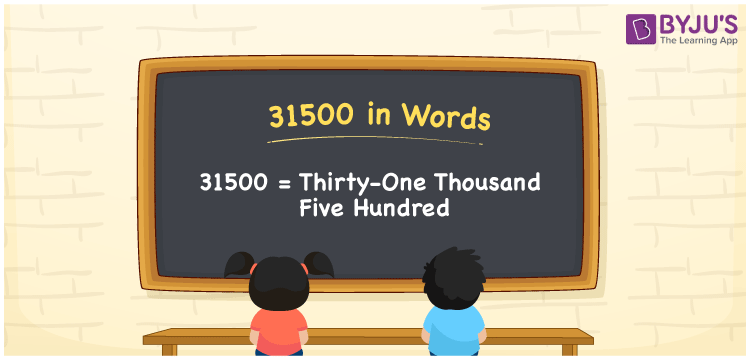# 31500 in Words

31500 in words can be written as Thirty-one Thousand Five Hundred. The concept of how to count numbers and its conversion into words is discussed briefly. If you buy a refrigerator for Rs. 31500 at the appliances store, then you can say that “I bought a refrigerator for Thirty-one Thousand Five Hundred Rupees at the appliances store”. Here you will learn how numbers in words concept is important in our day to day activities. Therefore, the number 31500 in words can be read as “Thirty-one Thousand Five Hundred”.

 31500 in words Thirty-one Thousand Five Hundred Thirty-one Thousand Five Hundred in Numbers 31500

## 31500 in English Words## How to Write 31500 in Words?

You will learn in brief about the place value chart of the number 31500 here. There are five digits in 31500. The table below will help you understand the place value for five digits in a clear cut manner.

 Ten Thousands Thousands Hundreds Tens Ones 3 1 5 0 0

The number 31500 in expanded form is:

3 x Ten Thousand + 1 x Thousand + 5 × Hundred + 0 × Ten + 0 × One

= 3 x 10000 + 1 x 1000 + 5 × 100 + 0 × 10 + 0 × 1

= 30000 + 1000 + 500

= 31500

= Thirty-one Thousand Five Hundred

Therefore, 31500 in words is written as Thirty-one Thousand Five Hundred.

31500 is a natural number that precedes 31501 and succeeds 31499.

31500 in words – Thirty-one Thousand Five Hundred

Is 31500 an odd number? – No

Is 31500 an even number? – Yes

Is 31500 a perfect square number? – No

Is 31500 a perfect cube number? – No

Is 31500 a prime number? – No

Is 31500 a composite number? – Yes

## Frequently Asked Questions on 31500 in Words

Q1

### How to write 31500 in words?

31500 can be written as “Thirty-one Thousand Five Hundred” in words.
Q2

### Find the value of 32000 minus 500.

The value of 32000 minus 500 is 31500. Therefore, 31500 in words is Thirty-one Thousand Five Hundred.
Q3

### Is 31500 an odd or even number?

31500 is an even number as it is completely divisible by 2.
31500/2 = 15750